# Two identical masses, one at rest and the other moving, undergo elastic

Two identical masses, one at rest and the other moving, undergo elastic oblique collision. Prove that they will move at right angles to each other after collision?

Let the equal masses be m and their initial velocities be u and zero respectively.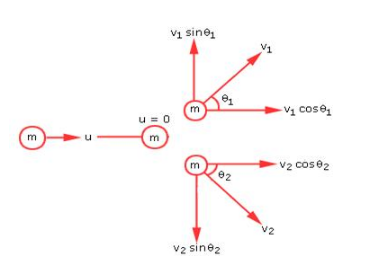Since momentum is a vector, according to conservation of momentum we have
mu = mv1cosθ1 + mv2cosθ2
i.e., u = v1cosθ1 + v2cosθ2 (i)
and 0 = v1sinθ1 - v2sinθ2 (ii)
Being an elastic collision, kinetic energy is also conserved.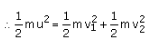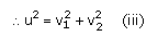Squaring and adding (i) and (ii), we get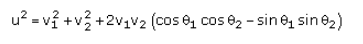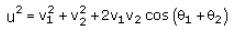Using (iii) in the above equation, we have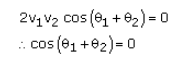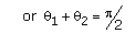i.e., the masses move at right angles after the collision.